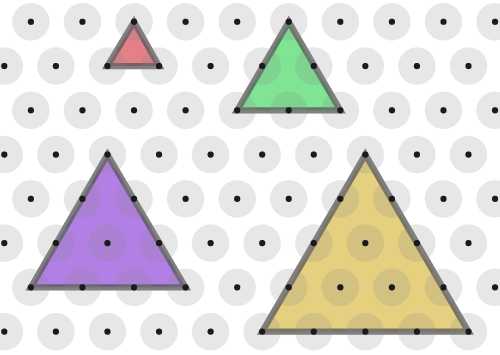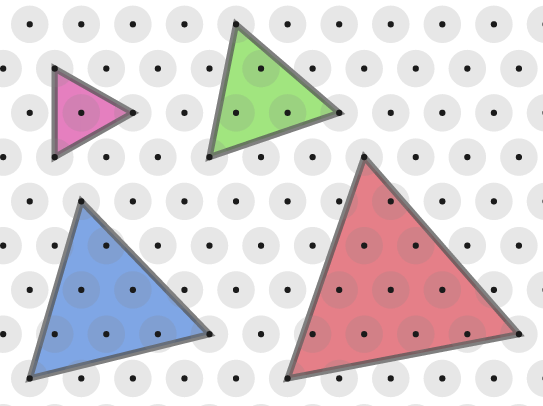#### You may also like### At a Glance

The area of a regular pentagon looks about twice as a big as the pentangle star drawn within it. Is it?### Six Discs

Six circular discs are packed in different-shaped boxes so that the discs touch their neighbours and the sides of the box. Can you put the boxes in order according to the areas of their bases?### Equilateral Areas

ABC and DEF are equilateral triangles of side 3 and 4 respectively. Construct an equilateral triangle whose area is the sum of the area of ABC and DEF.

# Of All the Areas

##### Age 14 to 16Challenge Level

This problem follows on from Isometric Areas and More Isometric Areas.
You may wish to print off some isometric paper or use the isometric dotty grid environment for this problem.

When working on an isometric grid, we can measure areas in terms of equilateral triangles instead of squares.

Here are some equilateral triangles.If the area of the smallest triangle is 1 unit, what are the areas of the other triangles?

Can you see a relationship between the area and the length of the base of each triangle?

Will the pattern continue?
Can you explain why?

All the triangles in the first image had horizontal bases, but it is also possible to draw "tilted" equilateral triangles.These triangles all have a "tilt" of 1.

Can you convince yourself that they are equilateral?

Can you find their areas?

Take a look at the hint for ideas on how to get started.

Can you find a rule to work out the area of any equilateral triangle with a "tilt" of 1?
Can you explain why your rule works?

What about areas of triangles with a "tilt" of 2?
What about areas of triangles with other "tilts"?